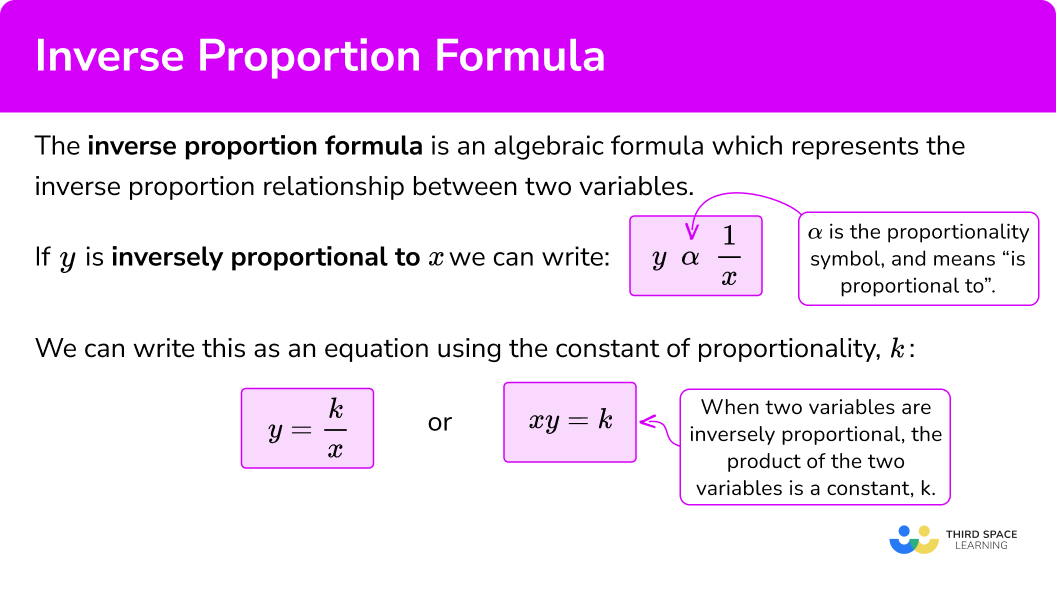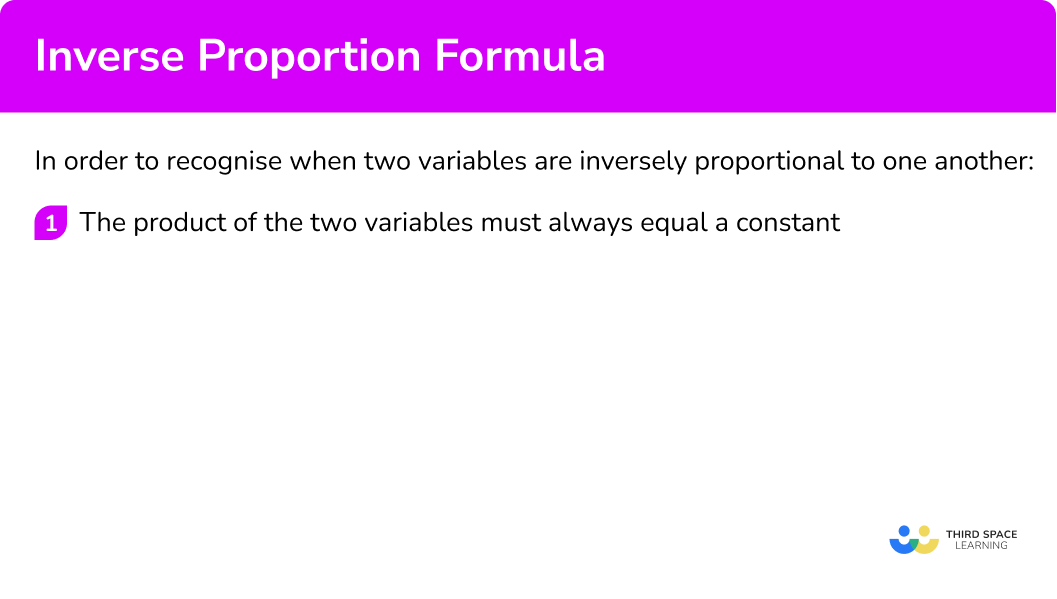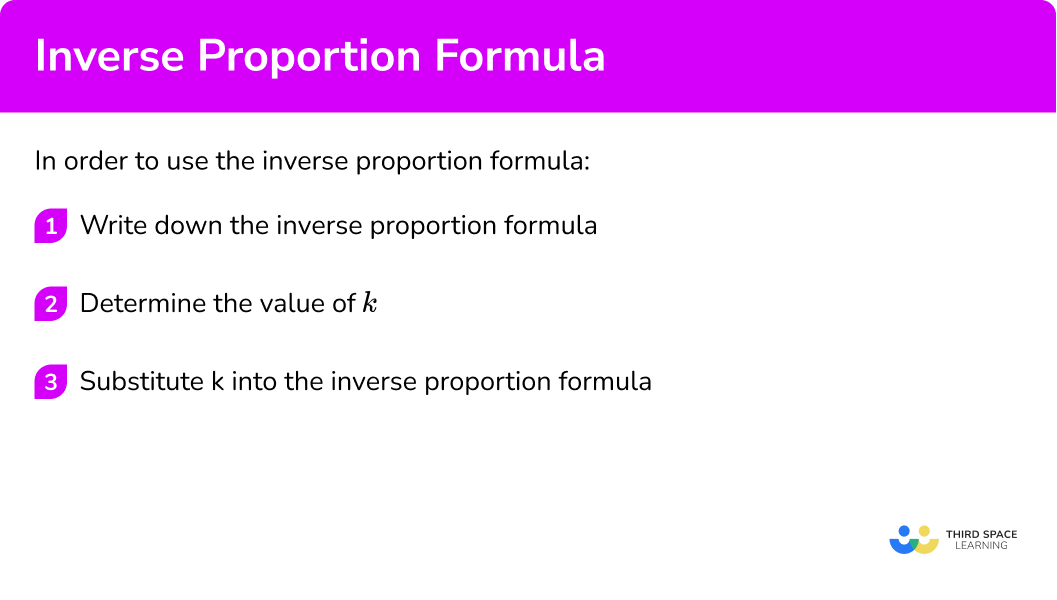# Inverse Proportion Formula

Here we will learn about inverse proportion formulas, including what the inverse proportion formulas are and how to interpret them.

There are also inverse proportion formula worksheets based on Edexcel, AQA and OCR exam questions, along with further guidance on where to go next if you’re still stuck.

## What is the inverse proportion formula?

The inverse proportion formula is an algebraic formula which represents the inverse proportion relationship between two variables.

If the variables were x and y where y is inversely proportional to x, we can write the relationship using the proportionality symbol as

y\propto \frac{1}{x}.

If y is inversely proportional to x, the constant of proportionality k=xy.

Rearranging this equation to make y the subject, we have

y=\frac{k}{x}.

An inverse proportion formula can involve powers and roots.

For example, if the variables were x and y and y is inversely proportional to x^2 we can write the relationship using the proportionality symbol as

y\propto \frac{1}{x^2}.

This can be written as an equation using the constant of proportionality, k,

y=\frac{k}{x^2}.

To recognise when two variables are inversely proportional to one another, the product of the two variables will be a constant, k.

Inverse proportion is a relationship between two variables, it is sometimes known as indirect proportion or inverse variation. If two quantities are inversely proportional then as one quantity increases, the other decreases.

### What is the inverse proportion formula?## How to recognise when two variables are inversely proportional

In order to recognise when two variables are inversely proportional to one another:

1. The product of the two variables must always equal a constant.

### How to recognise when two variables are inversely proportional## Recognising inverse proportion examples

### Example 1: recognising an inverse proportion

Which of these equations indicate that y\propto \frac{1}{x}?

ABC D

1. The product of the two variables must always equal a constant

For A, we have the two variables x and y. If we multiply both sides by x so we have xy=\frac{x^2}{a}, \ xy is not a constant as \frac{x^2}{a} will change, depending on the value for x. So x and y are not inversely proportional for A.

For B, we have the two variables x and y. If we multiply both sides by x, we get xy=2. As the product of x and y always equals 2 (a constant value), x is inversely proportional to y for B.

For C, we have the two variables y and x^{2}. If we multiply both sides of the equation by x^{2}, we have x^{2}y=x^{4} which is not a constant as x^{4} will change as the value of x changes. This means that for C, y is not inversely proportional to x^{2}.

For D, we have the two variables y and x. If we multiply both sides by x, we have xy=2x^{2} and so these are not inversely proportional to one another.

B shows an inverse proportion.

### Example 2: recognising an inverse proportion

Which of these equations indicate that y is inversely proportional to x?

ABC D

For A, we have the two variables x^{3} and y. If we multiply both sides by x^{3} so we have x^{3}y=x^{6}, \ x^{3}y is not a constant as x^{6} will change, depending on the value for x. So x and y are not inversely proportional for A.

For B, we have the two variables x and y. If we multiply both sides by x, we have xy=\frac{x^{2}}{3}. This means that xy is not a constant as \frac{x^{2}}{3} will change, depending on the value for x. So x and y are not inversely proportional for B.

For C, we have the two variables x and y. Multiplying both sides by x, we have xy=3x^2 which is not a constant value and so y is not inversely proportional to x for C.

For D, we have the two variables x and y. Multiplying both sides by x, we have xy=3 which is a constant and so y is inversely proportional to x for D.

D shows an inverse proportion.

## How to use the inverse proportion formula

In order to use the inverse proportion formula:

1. Write down the inverse proportion formula.
2. Determine the value of \textbf{k} .
3. Substitute \textbf{k} into the inverse proportion formula.

### How to use the inverse proportion formula## Inverse proportion formula examples

### Example 3: determine the inverse proportion formula

Let y be inversely proportional to x. When y=5, \ x=6. By calculating the constant of proportionality, determine a formula for y in terms of x.

As y is inversely proportional to x, we can write y\propto\frac{1}{x} and so we have the formula y=\frac{k}{x} where k is the constant of proportionality.

We can find the constant of proportionality by substituting in the values we are given.

\begin{aligned} y&= \frac{k}{x} \\\\ 5 &= \frac{k}{6} \\\\ k&=5\times 6=30 \end{aligned}

As k=30, we have the equation

y=\frac{30}{x}.

### Example 4: determine the inverse proportion equation

y is inversely proportional to x^{3}.

When y=4, \ x=2.

Find a formula for y in terms of x.

y\propto\frac{1}{x^{3}} and so y=\frac{k}{x^{3}}.

As y=4 when x=2,

\begin{aligned} 4&=\frac{k}{2^{3}} \\\\ 4&=\frac{k}{8} \\\\ 4\times{8}&=k \\\\ k&=32 \end{aligned}

As y=\frac{k}{x^{3}} and k=32,

y=\frac{32}{x^{3}}.

### Example 5: constructing an inverse proportion formula

Let y be inversely proportional to x^{2}.

When y=10, \ x=2.

Find a formula for y in terms of x.

From the first sentence we can write down y\propto \frac{1}{x^2}.

We can therefore state

y=\frac{k}{x^2}

where k is the constant of proportionality.

We can find the constant of proportionality by substituting in the values we are given.

Here x=2 and y=10 .

\begin{aligned} y&= \frac{k}{x^2} \\\\ 10 &= \frac{k}{2^2} \\\\ 10&=\frac{k}{4} \\\\ k&=10\times 4=40 \end{aligned}

As k=40 we can write down the equation

y=\frac{40}{x^2}.

### Example 6: constructing an inverse proportion equation from a table

Use the information in the table below to determine the formula, given that b is inversely proportional to the square root of a.

As stated in the question, b\propto\frac{1}{\sqrt{a}} and so b=\frac{k}{\sqrt{a}} .

When a=4, \ b=1 .

\begin{aligned} 1&=\frac{k}{\sqrt{4}} \\\\ 1&=\frac{k}{2} \\\\ 1\times{2}&=k \\\\ k&=2 \end{aligned}

Note that we could have used any pair of values for a and b from the table.

Now that k=2, we have the inverse proportion formula

b=\frac{2}{\sqrt{a}}.

### Example 7: constructing and solving an inverse proportion equation from a worded problem

The number of people setting tables in a restaurant (p) is inversely proportional to the time taken to set all of the tables (t). It takes 6 people, 48 minutes to set up all of the tables in the restaurant.

How many minutes would it take to set up all of the tables with 10 people?

As stated in the question, p\propto\frac{1}{t} and so p=\frac{k}{t}.

When p=6, \ t=48 and so

\begin{aligned} 6&=\frac{k}{48} \\\\ 6\times{48}&=k \\\\ k&=288 \end{aligned}

Now that k=288, we have the inverse proportion formula

p=\frac{288}{t}.

We have the extra condition that we need to determine the number of minutes it would take 10 people to set up the tables. To do this, we need to substitute p=10 into the inverse proportion formula, and solve for t.

\begin{aligned} 10&=\frac{288}{t} \\\\ 10\times{t}&=288 \\\\ t&=\frac{288}{10}=28.8\text{ minutes} \end{aligned}

### Common misconceptions

• Direct proportion and inverse proportion

You will need to learn which formula is for which type of proportionality.

Direct proportion Inverse proportion

\hspace{0.5cm} y\propto x \hspace{3.5cm} y\propto \frac{1}{x}

\hspace{0.5cm} y=kx \hspace{3.35cm} y=\frac{k}{x}

• Check if there are any powers or roots involved

Most inverse proportional formulae just involve x, but they can involve powers such as x^2 or roots such as \sqrt{x}.

• Constant of proportionality

The constant of proportionality can be an integer (a whole number), but they can also be decimals or fractions. For example,

y=\frac{5}{2x}=\frac{5}{2}\times\frac{1}{x}=2.5\times\frac{1}{x}=\frac{2.5}{x} and so k=2.5.

## Related lessons on inverse proportion formula

Inverse proportion formula is part of our series of lessons to support revision on proportion. You may find it helpful to start with the main proportion lesson for a summary of what to expect, or use the step by step guides below for further detail on individual topics. Other lessons in this series include:

### Practice inverse proportion formula questions

1. Which of these equations does not indicate y\propto\frac{1}{x}?

y=\frac{2}{x}y=5xxy=8y=\frac{1}{3x}The incorrect equation is y=5x as xy=5x^{2} which is not a constant.

2. Which of these equations indicate y\propto\frac{1}{x}?

y=5xy=\frac{5}{x}y=\frac{x}{5}y=5x^2For y\propto\frac{1}{x}, \ k=xy. When y=\frac{5}{x}, \ xy=5 which is a constant and so this is the correct solution.

3. Which of these equations indicate y\propto\frac{1}{x^{2}}?

y=\frac{10}{x}y=10x^2y=\frac{10}{x^{2}}x^{2}y=\frac{10}{x}When y\propto\frac{1}{x^{2}}, \ x^{2}y=k.

For y=\frac{10}{x^{2}}, multiplying both sides by x^{2}, we have x^{2}y=10 and so this is the correct solution.

4. y is inversely proportional to x.

When y=2, \ x=8.

Find a formula for y in terms of x.

y=16xy=\frac{4}{x}y=\frac{x}{4}y=\frac{16}{x}y\propto\frac{1}{x} and so y=\frac{k}{x}.

As y=2 when x=8, \ y=\frac{k}{x}

\begin{aligned} 2&= \frac{k}{8} \\\\ k&=2\times 8=16 \end{aligned}

So the equation is y=\frac{16}{x}.

5. y is inversely proportional to x.

When y=10, \ x=5.

Find a formula for y in terms of x.

y=2xy=\frac{50}{x}y=50xy=\frac{2}{x}Write down the relationship between y and x and substitute the given values to find k, the constant of proportionality.
As y\propto\frac{1}{x} and so y=\frac{k}{x}.

As y=10 when x=5,

\begin{aligned} 10 &= \frac{k}{5} \\\\ k&=10\times{5}=50 \end{aligned}

So the equation is y=\frac{50}{x}.

6. y is inversely proportional to \sqrt{x}.

When y=2, \ x=9.

Find a formula for y in terms of \sqrt{x}.

y=\frac{6}{\sqrt{x}}y=\frac{6}{x^2}y=\frac{3}{\sqrt{x}}y=6\sqrt{x}As y\propto\frac{1}{\sqrt{x}}, \ y=\frac{k}{\sqrt{x}}
As y=2 when x=9,

\begin{aligned} 2&= \frac{k}{\sqrt{9}}\\\\ 2 &= \frac{k}{3}\\\\ k&=2\times 3=6 \end{aligned}

So the equation is y=\frac{6}{\sqrt{x}}.

### Inverse proportion formula GCSE questions

1. Let y be inversely proportional to x, where k is a constant.

Identify the correct equation.

Equation AEquation B Equation C Equation D

\hspace{.5cm} y=\frac{k}{x} \hspace{1.5cm} y=kx \hspace{1.4cm} y=k-x \hspace{1.4cm} y=\frac{x}{k}

(1 mark)

Equation A or y=\frac{k}{x} .

(1)

2. Let y be inversely proportional to x, When y=9, \ x=3.

(a) Find the equation for y in terms of x.

(b) Find the value of x when y=10.

(5 marks)

(a)

y=\frac{k}{x}

(1)

k=9 \times 3=27

(1)

y=\frac{27}{x}

(1)

(b)

10=\frac{27}{x} or x=27\div 10 .

(1)

x=2.7

(1)

3. The number of workers (w) building a pyramid is inversely proportional to the time taken to build the pyramid (t).

It takes 9 men, 5 years to build a pyramid.

How many workers would be needed to build the same pyramid in 3 years?

(3 marks)

w=\frac{k}{t} or 9=\frac{k}{5}

(1)

k=45

(1)

w=\frac{45}{3}=15

(1)

## Learning checklist

You have now learned how to:

• Solve problems involving inverse proportion, including algebraic representations

## Still stuck?

Prepare your KS4 students for maths GCSEs success with Third Space Learning. Weekly online one to one GCSE maths revision lessons delivered by expert maths tutors.

Find out more about our GCSE maths tuition programme.